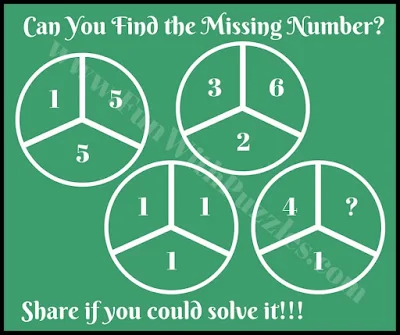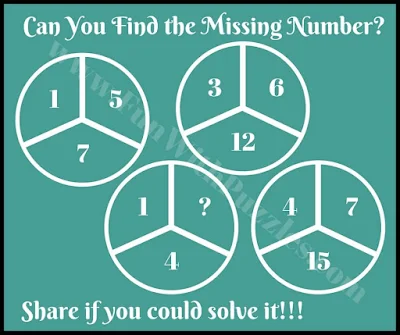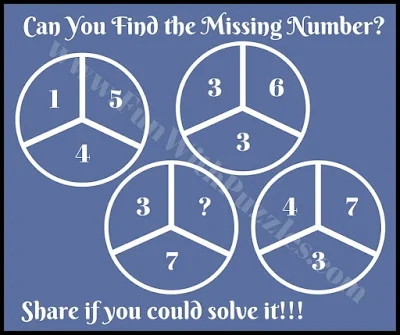It's been a long time since we published Maths Brain Teasers. Our previous post on Maths Puzzle was "Interesting and Confusing Math Brain Test" which contained different Maths Puzzles which were used with different geometrical shapes. E.g. it contained Maths Puzzles with Square shapes in which four numbers were given. It also contained Three Squared Math Puzzles in which 3 numbers were given. Also, it contained Maths Brain Teaser in Triangular as well as Circle Shapes. One thing that was common in all these Math Brain Teasers is that they contain a certain set of numbers that share a relationship with each other. One has to find this relationship among these numbers and then solve it for the missing number.

## How to Solve Circle Maths Puzzles

In these 'Cool Maths Quiz for Kids', four circles are given in each puzzle picture. Each circle contains three numbers. These three numbers share one Mathematical relationship with each other. One has to find this pattern or relationship among these three numbers and then has to solve each Maths Brain Teaser for the missing number which will replace the question mark.1. What will replace the question mark?2. Calculate the value of the Missing Number?3. What is the value of the Missing Number?4. Can you solve this Simple Maths Puzzle?5. Solve this Maths Riddle to find the missing number?

If you love to solve Maths Brain Teaser, then you can check out the following Maths Puzzles.

1. 4

2. 17

3.  2

4.  3

5. 10